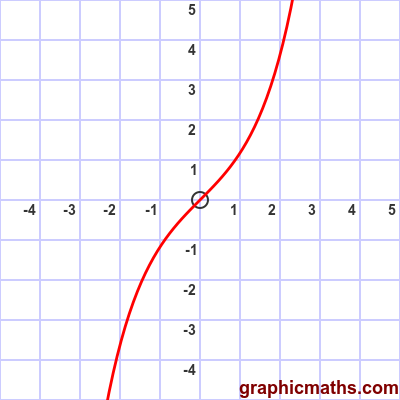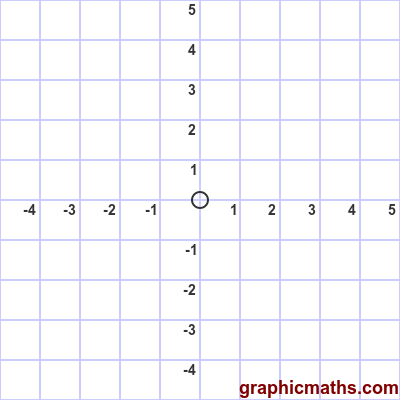# sinh function

Martin McBride, 2020-09-10
Tags sinh
Categories hyperbolic functions pure mathematics

The sinh function is a hyperbolic function. It is also known as the hyperbolic sine function.

## Equation and graph

The sinh function is defined as:

$$\sinh{x} = \frac{e^{x}-e^{-x}}{2}$$

Here is a graph of the function:## sinh as average of two exponentials

The sinh function can be interpreted as the average of two functions, $e^{x}$ and $-e^{-x}$. This animation illustrates this:## Other forms of the equation

If we multiply the top and bottom of the original equation for the sinh function by $e^{x}$ we get:

$$\sinh{x} = \frac{e^{2x}-1}{2e^{x}}$$

Alternatively, if we multiply the top and bottom of the original equation for the sinh function by $e^{-x}$ we get:

$$\sinh{x} = \frac{1-e^{-2x}}{2e^{-x}}$$

These alternative forms are sometimes useful.